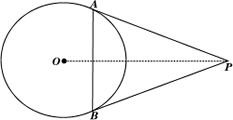Visitors Online: 0 | Saturday 07th December 2019CBSE Guess > Papers > Important Questions > Class X > 2014 > Mathematics > Math-index > Mathematics By Mr. Ajai Kumar Shukla CBSE CLASS X Mathematics MATHEMATICS How many lead balls, each of radius 1cm, can be made from a sphere ofradius 8cm? Find the probability that a number selected at random from the numbers 1 to 25 is not a prime number when each of the given numbers is equally likely to be selected. If the point P(x, y) is equidistant from the points A (5, 1) and B (–1, 5), prove that 3x = 2y. There are 35 students in a class of whom 20 are boys and 15 are girls. From these students one is chosen at random. What is the probability that the chosen student is a (i) boy (ii) girl? A card is drawn at random from a well-shuffled pack of 52 cards. Find the probability that the card drawn is neither a red card nor a queen. The first term of an AP is 5, the last term is 45 and the sum is 400. Find the number of terms and the common difference. Prove that the points A(–3, 0), B(1, –3) and C(4, 1) are the vertices of an isosceles right-angled triangle. Find the area of this triangle. From a point P, two tangents PA and PB are drawn to a circle C(O, r). If OP = 2r, show that APB is equilateral.Metallic spheres of radii 6cm, 8cm and 10cm, respectively, are melted to form a single solid sphere. Find the radius of the resulting sphere. The angle of elevation of the top of a building from the foot of the tower is 30º and the angle of elevation of the top of the tower from the foot of the building is 60º. If the tower is 50m high, find the height of the building. From the top of a 7m high building, the angle of elevation of the top of a cable tower is 60º and the angle of depression of its foot is 45º. Determine the height of the tower. A solid is composed of a cylinder with hemispherical ends. If the whole length of the solid is 104 cm and the radius of each of its hemispherical ends is 7 cm, find the cost of polishing its surface at the rate of Rs. 10 per dm2. CBSE Important Questions Class X Mathematics By : Mr. Ajai Kumar Shukla Submitted By : Mr. Ajai Kumar Shukla, RACHNA TUTORIALS, 6/1003 JANKIPURAM VISTAR LUCKNOW Mobile : +91 - 9415467421 Email Id : [email protected]